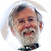Skip to main content

# Human population growth and its impact on genetic variationPublished on April 21, 2015   34 min
• Add to
• Review

#### Other Talks in the Series: Human Population Genetics II

0:00
Hi, my name is Andy Clark, and I would like to tell you about the human population growth and its impact on genetic variation.
0:10
We're going to begin with some simple population genetics to get an idea of what we expect to be the behavior of genetic variation in a population of different sizes. First we're going to start with a particular model called an infinite alleles model, and this model describes the balance between the input of genetic variation by mutation, and the loss of that variation by random genetic drift. This model predicts that the amount of heterozygosity, H in the population at equilibrium will be theta divided by theta plus one. I'll spare you the derivation of that, but this is an essential conclusion from this infinite alleles model. Theta is the population mutation rate, and theta is equal to four times the effective population size times the mutation rate. I'll be talking a little bit more about what that term "effective population size" is in just a moment. As I mentioned, H is the heterozygosity of the population or the probability that when you draw two copies of a gene, they will be different from each other in a sample from that population.
1:20
If we're talking about DNA sequences, we don't want to replace that term H, the heterozygocity, by a term that we very easily measure when we get DNA sequences from individuals, and that's this term pi. Pi is also referred to as the nucleotide diversity, or the average probability that any pair of nucleotides across two different copies of a gene are going to be different than each other. Now, you notice that I just replaced the H with pi, and all that we're doing here is considering the value of theta for the mutation rate per the nucleotide site in the genome. Now if theta then is very small, which it will be considering the mutation rate per single nucleotide in the genome, that's again on the order of ten to the minus eighth or so, then that denominator term theta plus one is going to be very close to one itself, because theta is so small. So we can just remove that denominator and say pi is approximately equal to theta. And as we said before, theta is equal to four times any times new. So in this equilibrium case, the model predicts that the nucleotide diversity should simply equal four and new approximately.
Hide